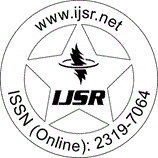International Journal of Science and Research (IJSR)
Call for Papers | Fully Refereed | Open Access | Double Blind Peer Reviewed

Downloads: 129 | Views: 196 | Weekly Hits: ⮙1 | Monthly Hits: ⮙1

Research Paper | Mechanical Engineering | India | Volume 7 Issue 6, June 2018

# Mathematical Analysis of Static and Dynamic Forces of Spur Gear Drive with High Contact Ratio

Sateesh Kumar Singh | Shiv Kumar Tripathi 

Abstract: In this work, the procedure used for determination of the load acting on the various teeth in mesh of spur gear drive with high contact ratio is mathematical using mathematical expressions. In this procedure, it is assumed that in all circumstances the tooth deflections are equal at every pairs of contact, either it is two pairs or three pairs. Also it is assumed that summation of normal loads shared by each of two or three pairs of contact is equal to peak normal load. In this, a mathematical method based on torsional vibration of gears is used to determine the dynamic load of spur gear drive having high contact ratio. In the duration of meshing of gear, the dynamic load variations are determined using this. For calculations of dynamic load, it is required to determine variable stiffness, which is determined by using mathematical expressions. In the study of tooth stiffness calculations, various factors like bending compression, shear compression, axial compression and Hertzian contact deflections are considered. Friction amongst various teeth in contact and viscous damping are also taken in consideration in this analysis. In the analysis two elastic bodies is used which is held in contact by forces normal to the area of contact together with tangential frictional force is used for calculation of contact stresses. The variations in contact stresses during meshing are also determined. It is also found that the dynamic load which is acting on the gear tooth is greatly influenced by the operating speed. Due to change in operating speed, the value and location of peak dynamic load also changes.

Keywords: Mesh stiffness, Dynamic load, High contact ratio, Spur gears

Edition: Volume 7 Issue 6, June 2018,

Pages: 1549 - 1552